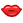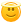# variable not updating -c++-

so i am making this code just for fun and in need to update the variable "incorrect"so at the end of the program i can see if "incorrect" is >= 3 but incorrect ,for some reson, is always 0

note:i have not finished the code btw

#include <iostream>

using namespace std;

void question_1()
{
cout<<"what is (5+5) ÷ 10? :"<<endl;
cout<<"correct!!! But that was an easy one so that is what is to be expected,now onto the next question."<<endl;
}else{
cout<<"\a\a\a\a\a\a\a\a\a\a\a\a\a\a\a\a\a\a\a";
cout<<"ha stupid human the answer was 1 that was sooooooooo obvious how did you get that wrong well you can only get 2 more wrong before you die\n";
int incorrect;
incorrect=0;
incorrect=incorrect + 1;
}

}

void question_2()
{
cout<<"now,second question what is 5!\n";
cout<<"that is correct thank you for not being stupid\n";
}else{
cout<<"\a\a\a\a\a\a\a\a\a\a\a\a\a\a\a";
cout<<"if you didn't know ,moron, 5! is equal to 5 facttorial witch is 2x3x4x5=120 daah";
}
}
int main()
{
cout<<"greetings,I am T.E.S.T. witch stands for 'Termanating Every Student that cant pass this Test'"<<endl;
cout<<"you will be asked 20 questions if you get 3 wrong you will be termenated"<<endl<<endl<<endl<<endl<<endl;
question_1();
question_2();
}

*************************************************************************************************

so if you can help in any way it will be appreciated.

+1 vote
answered Sep 29, 2020 by (77,930 points)
selected Sep 29, 2020 by Ike Roland

"but incorrect ,for some reson, is always 0"

That is not true. The variable incorrect changes its value on the call

`incorrect=incorrect + 1;`

Please, notice that the scope of the variable incorrect is limited to the else branch in function question_1().

That is, after you leave the else branch, the variable ceases to exist. The next time you call question_1() and enter the same else branch, the variable is recreated with a new value: 0 (which will be incremented by 1).

I think what you wanted to do here is declare incorrect as a global variable outside of the scope of your functions.

```#include <iostream>

using namespace std;

static int incorrect = 0;

void question_1()
// ...```
+1 vote
answered Sep 29, 2020 by (10,500 points)

Dude whatsup with the aggressive wording in the code {terminate ,stupid , die }? You seem very tense ,distressed ,almost about to explode if the code wont compile. OR I am "reading" too much from the code .

Take you time with the learning to write code ,it may look intimidating(even absurd) initally ,but its not that difficult if you give it enuf time(gradually ofc , even I learn something new after almost 5 years of work exp) .

answered Oct 12, 2020 by (1,080 points)

This is C++, not C. But you wrote c in tags for some reason.

answered Oct 14, 2020 by (220 points)
you could declare you variable incorrect in the main program and simply update it with a return value of 1 "incorrect" or 0 "correct" from the called functions. See example below:

#include <iostream>

using namespace std;

int question_1()
{
cout<<"what is (5+5) ÷ 10? :"<<endl;
cout<<"correct!!! But that was an easy one so that is what is to be expected,now onto the next question."<<endl;
return 0;
}else{
cout<<"\a\a\a\a\a\a\a\a\a\a\a\a\a\a\a\a\a\a\a";
cout<<"ha stupid human the answer was 1 that was sooooooooo obvious how did you get that wrong well you can only get 2 more wrong before you die\n";
return 1;
}
}

int question_2()
{
cout<<"now,second question what is 5!\n";
cout<<"that is correct thank you for not being stupid\n";
return 0;
}else{
cout<<"\a\a\a\a\a\a\a\a\a\a\a\a\a\a\a";
cout<<"if you didn't know ,moron, 5! is equal to 5 facttorial witch is 2x3x4x5=120 daah";
return 1;
}
}
int main()
{
int incorrect=0;
cout<<"greetings,I am T.E.S.T. witch stands for 'Termanating Every Student that cant pass this Test'"<<endl;
cout<<"you will be asked 20 questions if you get 3 wrong you will be termenated"<<endl<<endl<<endl<<endl<<endl;
incorrect+=question_1();
incorrect+=question_2();
cout<<"\n# incorrect = " << incorrect << endl;
}